# Texas Go Math Grade 5 Unit 1 Answer Key Number and Operations: Place Value and Operations

Refer to our Texas Go Math Grade 5 Answer Key Pdf to score good marks in the exams. Test yourself by practicing the problems from Texas Go Math Grade 5 Unit 1 Answer Key Number and Operations: Place Value and Operations.

## Texas Go Math Grade 5 Unit 1 Answer Key Number and Operations: Place Value and Operations

Show What You Know

Meaning of Division: Use counters to solve.

Question 1.
Divide 18 counters into groups of 3. How many groups are there?
___________ groups
It is given that
Divide 18 counters into groups of 3
Now,
Let the number of counters be c
Let the number of groups divided be g
Now,
From the given information,
The total number of groups = $$\frac{c}{g}$$
= $$\frac{18}{3}$$
= 6 groups
Hence, from the above,
We can conclude that
The total number of groups present are: 6 groups

Question 2.
Divide 21 counters into groups of 7. How many groups are there?
___________ groups
It is given that
Divide 21 counters into groups of 7
Now,
Let the number of counters be c
Let the number of groups divided be g
Now,
From the given information,
The total number of groups = $$\frac{c}{g}$$
= $$\frac{21}{7}$$
= 3 groups
Hence, from the above,
We can conclude that
The total number of groups present are: 3 groups

Place Value of Decimals: Write the word form and the expanded form for each.

Question 3.
3.4
The given number is: 3.4
Now,
We know that,
“Expanded form” means writing the number so you show the value of each digit. “Word form” means writing the number with words instead of numerals.
So,
The representation of the given number in the Word form is: Three and Four Tenths
So,
The representation of the given number in the Expanded form is: 3 + 0.4
Hence, from the above,
We can conclude that
The representation of the given number in the Word form is: Three and Four Tenths
The representation of the given number in the Expanded form is: 3 + 0.4

Question 4.
2.51
The given number is: 2.51
Now,
We know that,
“Expanded form” means writing the number so you show the value of each digit. “Word form” means writing the number with words instead of numerals.
So,
The representation of the given number in the Word form is: Two and Fifty-one hundredths
So,
The representation of the given number in the Expanded form is: 2 + 0.5 + 0.01
Hence, from the above,
We can conclude that
The representation of the given number in the Word form is: Two and Fifty-one hundredths
The representation of the given number in the Expanded form is: 2 + 0.5 + 0.01

Estimate Quotients: Using Compatible Numbers

Estimate the quotients.

Question 5.The given decimal expression is: 312 ÷ 2
Now,
By using the Long Division,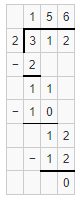Hence, from the above,
We can conclude that
The quotient for the given division expression is: 156

Question 6.The given division expression is: 189 ÷ 4
Now,
By using the Long Division,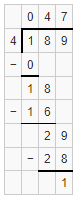Hence, from the above,
We can conclude that
The quotient for the given division expression is: 47 R 1

Question 7.The given division expression is: 603 ÷ 6
Now,
By using the Long Division,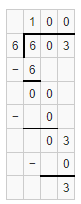Hence, from the above,
We can conclude that
The quotient for the given division expression is: 100 R 3

Question 8.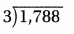The given division expression is: 1,788 ÷ 3
Now,
By using the Long Division,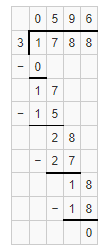Hence, from the above,
We can conclude that
The quotient for the given division expression is: 596

Vocabulary Builder

Visualize It

Use the ✓ words to complete the tree map.The completed tree map is:Understand Vocabulary

Read the description. Which word do you think is described?

Question 1.
One of one hundred equal parts _______________.
We know that,
One of one hundred equal parts is called as “Hundredth”
Hence, from the above,
We can conclude that
The suitable word that describes the given description is: Hundredth

Question 2.
The value of each digit in a number based on the location of the digit _______________.
We know that,
The value of each digit in a number based on the location of the digit is called as “Place-Value”
Hence, from the above,
We can conclude that
The suitable word that describes the given description is: Place-Value

Question 3.
To replace a number with one that is simpler and is approximately the same size as the original number _______________
We know that,
To replace a number with one that is simpler and is approximately the same size as the original number is called as “Round”
Hence, from the above,
We can conclude that
The suitable word that describes the given description is: Round

Question 4.
A property that states that multiplying a sum by a number is the same as multiplying each addend in the sum by the number then adding the products _______________.
We know that,
A property that states that multiplying a sum by a number is the same as multiplying each addend in the sum by the number then adding the products is called as “Distributive Property”
Hence, from the above,
We can conclude that
The suitable word that describes the given description is: Distributive Property

Question 5.
One of ten equal parts _______________.
We know that,
One of ten equal parts is called as “Tenth”
Hence, from the above,
We can conclude that
The suitable word that describes the given description is: Tenth

Question 6.
A familiar number used as a point of reference _______________.
We know that,
A familiar number used as a point of reference is called as “Benchmark”
Hence, from the above,
We can conclude that
The suitable word that describes the given description is: Benchmark

Question 7.
One of one thousand equal parts _______________.
We know that,
One of one thousand equal parts is called as “Thousandth”
Hence, from the above,
We can conclude that
The suitable word that describes the given description is: Thousandth

Question 8.
Operations that undo each other _______________.
We know that,
Operations that undo each other are called as “Inverse operations”
Hence, from the above,
We can conclude that
The suitable word that describes the given description is: Inverse operations

Reading When you read a story, you can look at the illustrations to help you visualize, or picture, what is happening. To visualize math, it can be helpful to show the information and numbers in a word problem in a different way.

United States Olympic athlete Brian Martin, from Palo Alto, CA, has won 2 medals ¡n the luge event. Which of his practice times was better: forty-seven and sixty-nine thousandths seconds or forty-seven and two hundredths seconds?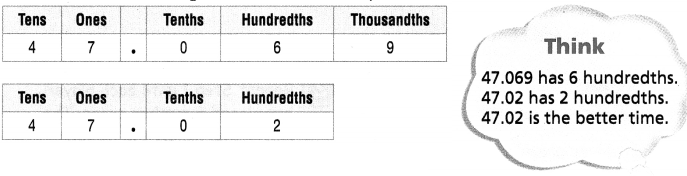Writing Work with a partner to visualize the numbers in different ways. Here are four possibilities:

• Use place-value models.
• Use standard form.
• Use a number line.

Which way do you prefer to use to help you visualize the numbers and solve the problem? Explain.It is given that
United States Olympic athlete Brian Martin, from Palo Alto, CA, has won 2 medals ¡n the luge event
Now,
It is given that
The visualization the numbers will be done in different ways. The possibilities for the visualization are:
a. Use place-value models.
b. Use standard form.
c.Use a number line.
Now,
From the given possibilities,
The place-value models would be preferred to visualize the numbers
Now,
The given place-value models are;Now,
From the given place-value models,
We can observe that
The hundredths and thousandths place values are different
Now,
We can observe that
6 > 2
9 > 0 (Since there is no thousand place value in the second place-value table, assume thousandth’s position as zero)
Hence, from the above,
We can conclude that
We prefer Place-value models to visualize the problem
47.069 practice time was better for Brian Martin

Digit Challenge
Object of the Game Use your number cards to make the greatest number.

Materials

• Number/Symbol Cards: 4 sets labeled 0-9
• Strip of paper (1 per player, divided into 6 sections to represent the digits in the thousands, hundreds, tens, ones. tenths, and hundredths)

Set Up
Shuffle all the number cards and place them face down in a stack. Each player has a 6-section strip of paper.
Number of Players 2-4

How to Play

1. Each player draws a number card and places t on his or her strip of paper. Once placed, the card cannot be moved.
2. Repeat Step 1 until each player has placed 6 number cards. The player who makes the number with the greatest value scores one point.
3. Return all the cards to the stack and shuffle. Repeat steps 1-2, The first player to score a total of 10 points is the winner,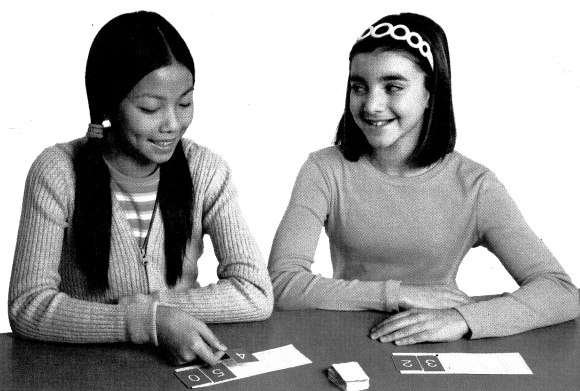Scroll to Top